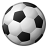# calculating raster pixel area for each individual raster pixel

2335
0
09-27-2013 07:53 AMNew Contributor
Hi

I am currently trying to calculate population sum for a raster layer which is in density per square kilometer for the entire earth's surface. Since raster pixels are not the same and change based on the latitude, I would like to calculate the individual raster cell area so I can then multiply population density per km by the total cell area. Ideally, I would like to assign this cell area value to the raster cell so I can multiply population density per km by the cell area to get total sum of population in that cell.

My question to put it simply is how do I calculate individual cell sizes of a raster and then assign it to it's pixel. (I am using  the Projected Coordinate System: WGS 1984 Web Mercator Auxiliary Sphere)

Thanks
Tags (3)
0 Replies